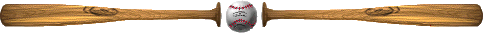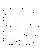# World Series Analytical Solution

##### (if the probability NL wins an individual game = 1/2)

# of games to win the seriesexplanationprobability

0

0

0

# 4

##### In order for the series to end after four games, one team must win the first four games in a row.
(1/2)(1/2)(1/2)(1/2) + (1/2)(1/2)(1/2)(1/2) = 2/16 = 1/8 = 0.1250

# 5

##### In order for the series to end in 5 games one team must win exactly 3 out of the first 4 games (in any order) and then win the fifth game.
C(4,3) (1/2)(1/2)(1/2)(1/2)(1/2) + C(4,3) (1/2)(1/2)(1/2)(1/2)(1/2) = 8/32 = 1/4 = 0.2500

# 6

##### In order for the series to end in 6 games one team must win exactly 3 out of the first 5 games (in any order) and then win the sixth game.
C(5,3) (1/2)(1/2)(1/2)(1/2)(1/2)(1/2) + C(5,3) (1/2)(1/2)(1/2)(1/2)(1/2)(1/2) = 20/64 = 5/16 = 0.3125

# 7

##### In order for the series to end in 7 games one team must win exactly 3 out of the first 6 games (in any order) and then win the seventh game.
C(6,3) (1/2)(1/2)(1/2)(1/2)(1/2)(1/2)(1/2) + C(6,3) (1/2)(1/2)(1/2)(1/2)(1/2)(1/2)(1/2) = 40/128 = 5/16 = 0.3125

Now check these answers. Remember, the total probability of all possibilities must equal 1.

##### Total probability = 0 + 0 + 0 + 0.1250 + 0.2500 + 0.3125 + 0.3125 = 1

As you can see, these numbers do check out. Now we find the expected value, E(x), by multiplying each value by its respective probability and adding them all together:

##### E(x) = 1(0) + 2(0) + 3(0) + 4(0.1250) + 5(0.2500) + 6(0.3125) + 7(0.3125) = 0 + 0 + 0 + 0.5000 + 1.2500 + 1.8750 + 2.1875 = 5.8125

Thus, by averaging all the trials from your model, you should get something near 5.8125 as your answer.

## Questions:

1. Should the average of the trials from your model be exactly 5.8125?

2. Does an expected value of 5.8125 mean that we expect someone to win 0.8125 of a game?

Please send questions and comments to Jay Hill